Publicité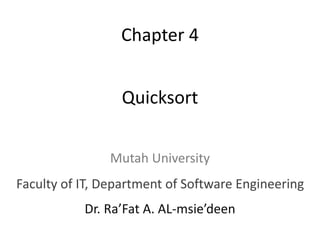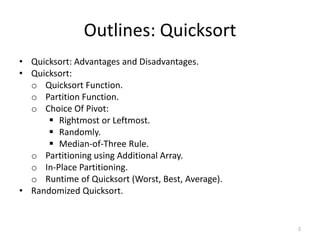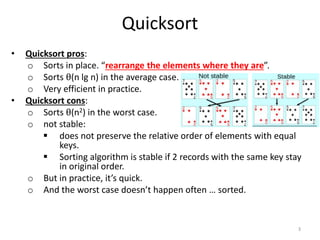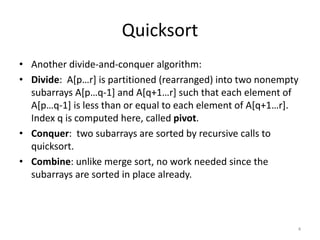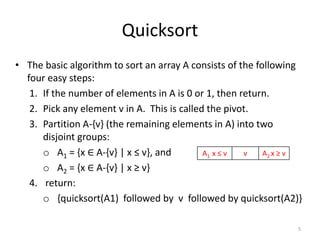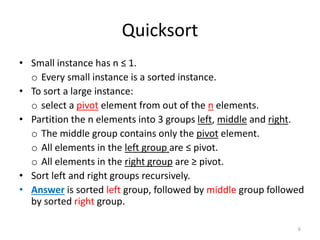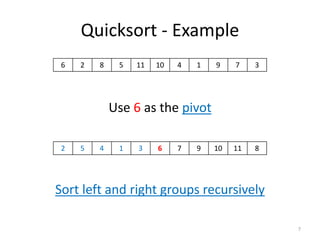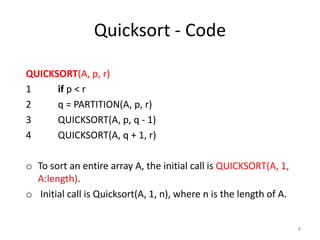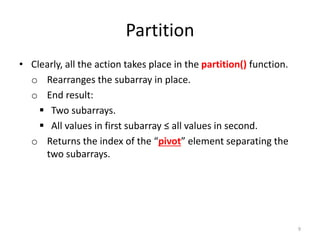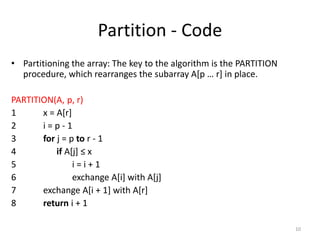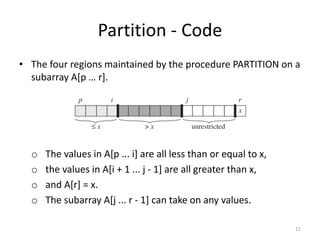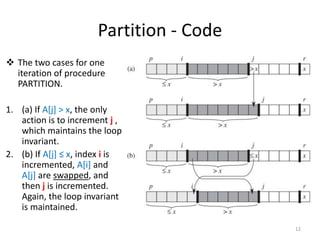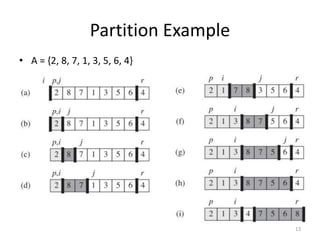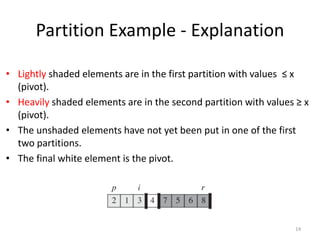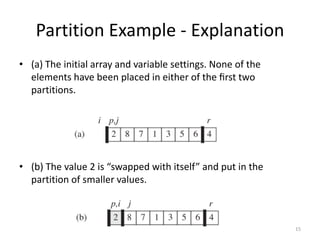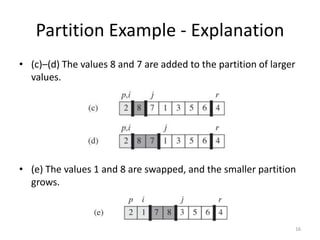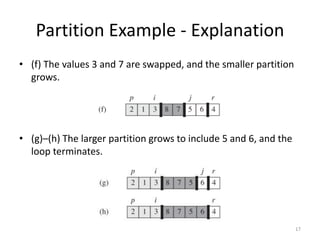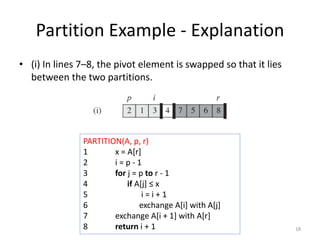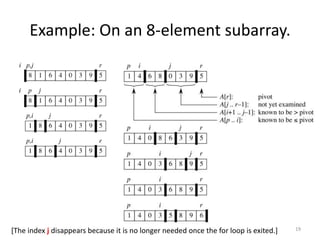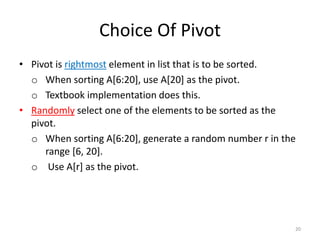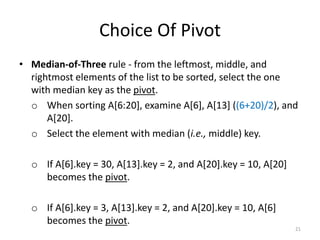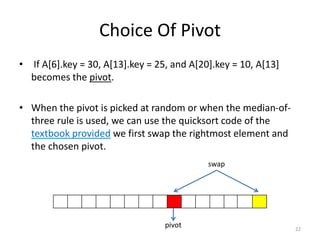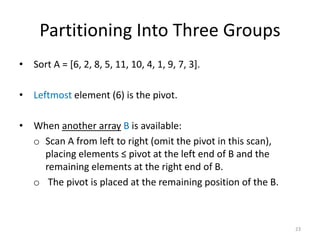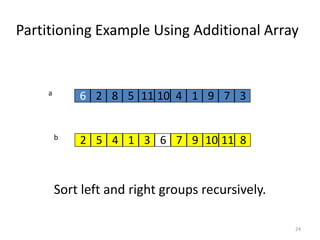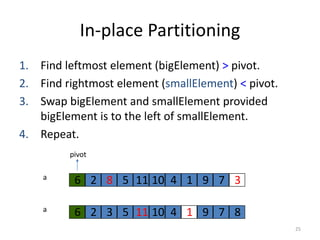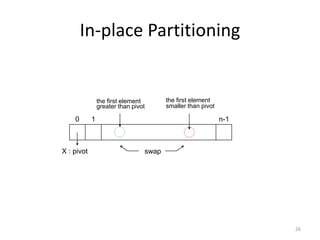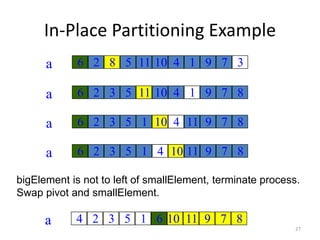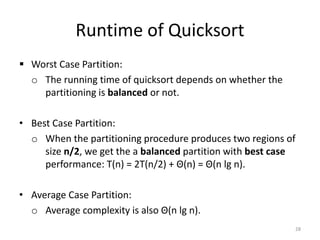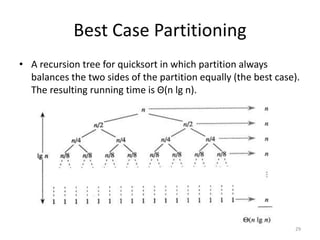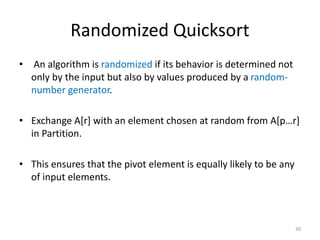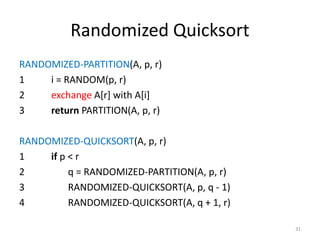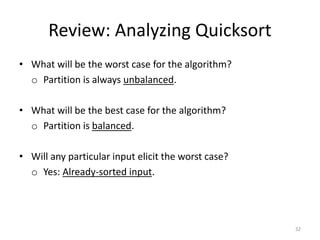1 sur 33
Publicité

### Algorithms - "quicksort"

1. Quicksort Dr. Ra’Fat A. AL-msie’deen Chapter 4 Mutah University Faculty of IT, Department of Software Engineering
2. Outlines: Quicksort • Quicksort: Advantages and Disadvantages. • Quicksort: o Quicksort Function. o Partition Function. o Choice Of Pivot:  Rightmost or Leftmost.  Randomly.  Median-of-Three Rule. o Partitioning using Additional Array. o In-Place Partitioning. o Runtime of Quicksort (Worst, Best, Average). • Randomized Quicksort. 2
3. Quicksort • Quicksort pros: o Sorts in place. “rearrange the elements where they are”. o Sorts θ(n lg n) in the average case. o Very efficient in practice. • Quicksort cons: o Sorts θ(n2) in the worst case. o not stable:  does not preserve the relative order of elements with equal keys.  Sorting algorithm is stable if 2 records with the same key stay in original order. o But in practice, it’s quick. o And the worst case doesn’t happen often … sorted. 3
4. Quicksort • Another divide-and-conquer algorithm: • Divide: A[p…r] is partitioned (rearranged) into two nonempty subarrays A[p…q-1] and A[q+1…r] such that each element of A[p…q-1] is less than or equal to each element of A[q+1…r]. Index q is computed here, called pivot. • Conquer: two subarrays are sorted by recursive calls to quicksort. • Combine: unlike merge sort, no work needed since the subarrays are sorted in place already. 4
5. Quicksort • The basic algorithm to sort an array A consists of the following four easy steps: 1. If the number of elements in A is 0 or 1, then return. 2. Pick any element v in A. This is called the pivot. 3. Partition A-{v} (the remaining elements in A) into two disjoint groups: o A1 = {x ∈ A-{v} | x ≤ v}, and o A2 = {x ∈ A-{v} | x ≥ v} 4. return: o {quicksort(A1) followed by v followed by quicksort(A2)} 5 A1 x ≤ v v A2 x ≥ v
6. Quicksort • Small instance has n ≤ 1. o Every small instance is a sorted instance. • To sort a large instance: o select a pivot element from out of the n elements. • Partition the n elements into 3 groups left, middle and right. o The middle group contains only the pivot element. o All elements in the left group are ≤ pivot. o All elements in the right group are ≥ pivot. • Sort left and right groups recursively. • Answer is sorted left group, followed by middle group followed by sorted right group. 6
7. Quicksort - Example Use 6 as the pivot Sort left and right groups recursively 7 6 2 8 5 11 10 4 1 9 7 3 2 5 4 1 3 6 7 9 10 11 8
8. Quicksort - Code QUICKSORT(A, p, r) 1 if p < r 2 q = PARTITION(A, p, r) 3 QUICKSORT(A, p, q - 1) 4 QUICKSORT(A, q + 1, r) o To sort an entire array A, the initial call is QUICKSORT(A, 1, A:length). o Initial call is Quicksort(A, 1, n), where n is the length of A. 8
9. Partition • Clearly, all the action takes place in the partition() function. o Rearranges the subarray in place. o End result:  Two subarrays.  All values in first subarray ≤ all values in second. o Returns the index of the “pivot” element separating the two subarrays. 9
10. Partition - Code • Partitioning the array: The key to the algorithm is the PARTITION procedure, which rearranges the subarray A[p … r] in place. PARTITION(A, p, r) 1 x = A[r] 2 i = p - 1 3 for j = p to r - 1 4 if A[j] ≤ x 5 i = i + 1 6 exchange A[i] with A[j] 7 exchange A[i + 1] with A[r] 8 return i + 1 10
11. Partition - Code • The four regions maintained by the procedure PARTITION on a subarray A[p … r]. o The values in A[p ... i] are all less than or equal to x, o the values in A[i + 1 ... j - 1] are all greater than x, o and A[r] = x. o The subarray A[j ... r - 1] can take on any values. 11
12. Partition - Code  The two cases for one iteration of procedure PARTITION. 1. (a) If A[j] > x, the only action is to increment j , which maintains the loop invariant. 2. (b) If A[j] ≤ x, index i is incremented, A[i] and A[j] are swapped, and then j is incremented. Again, the loop invariant is maintained. 12
13. Partition Example • A = {2, 8, 7, 1, 3, 5, 6, 4} 13
14. Partition Example - Explanation • Lightly shaded elements are in the first partition with values ≤ x (pivot). • Heavily shaded elements are in the second partition with values ≥ x (pivot). • The unshaded elements have not yet been put in one of the first two partitions. • The final white element is the pivot. 14
15. Partition Example - Explanation • (a) The initial array and variable settings. None of the elements have been placed in either of the ﬁrst two partitions. • (b) The value 2 is “swapped with itself” and put in the partition of smaller values. 15
16. Partition Example - Explanation • (c)–(d) The values 8 and 7 are added to the partition of larger values. • (e) The values 1 and 8 are swapped, and the smaller partition grows. 16
17. Partition Example - Explanation • (f) The values 3 and 7 are swapped, and the smaller partition grows. • (g)–(h) The larger partition grows to include 5 and 6, and the loop terminates. 17
18. Partition Example - Explanation • (i) In lines 7–8, the pivot element is swapped so that it lies between the two partitions. 18 PARTITION(A, p, r) 1 x = A[r] 2 i = p - 1 3 for j = p to r - 1 4 if A[j] ≤ x 5 i = i + 1 6 exchange A[i] with A[j] 7 exchange A[i + 1] with A[r] 8 return i + 1
19. Example: On an 8-element subarray. 19[The index j disappears because it is no longer needed once the for loop is exited.]
20. Choice Of Pivot • Pivot is rightmost element in list that is to be sorted. o When sorting A[6:20], use A as the pivot. o Textbook implementation does this. • Randomly select one of the elements to be sorted as the pivot. o When sorting A[6:20], generate a random number r in the range [6, 20]. o Use A[r] as the pivot. 20
21. Choice Of Pivot • Median-of-Three rule - from the leftmost, middle, and rightmost elements of the list to be sorted, select the one with median key as the pivot. o When sorting A[6:20], examine A, A ((6+20)/2), and A. o Select the element with median (i.e., middle) key. o If A.key = 30, A.key = 2, and A.key = 10, A becomes the pivot. o If A.key = 3, A.key = 2, and A.key = 10, A becomes the pivot. 21
22. Choice Of Pivot • If A.key = 30, A.key = 25, and A.key = 10, A becomes the pivot. • When the pivot is picked at random or when the median-of- three rule is used, we can use the quicksort code of the textbook provided we first swap the rightmost element and the chosen pivot. 22 swap pivot
23. Partitioning Into Three Groups • Sort A = [6, 2, 8, 5, 11, 10, 4, 1, 9, 7, 3]. • Leftmost element (6) is the pivot. • When another array B is available: o Scan A from left to right (omit the pivot in this scan), placing elements ≤ pivot at the left end of B and the remaining elements at the right end of B. o The pivot is placed at the remaining position of the B. 23
24. Partitioning Example Using Additional Array Sort left and right groups recursively. 24 6 2 8 5 11 10 4 1 9 7 3a b 2 85 11104 1 973 6
25. In-place Partitioning 1. Find leftmost element (bigElement) > pivot. 2. Find rightmost element (smallElement) < pivot. 3. Swap bigElement and smallElement provided bigElement is to the left of smallElement. 4. Repeat. 25 6 2 8 5 11 10 4 1 9 7 3a 6 6 2 3 5 11 10 4 1 9 7 8a 6 pivot
26. In-place Partitioning 26 X : pivot 0 1 n-1 swap the first element greater than pivot the first element smaller than pivot
27. In-Place Partitioning Example 27 6 2 8 5 11 10 4 1 9 7 3a 6 8 3 6 2 3 5 11 10 4 1 9 7 8a 6 11 1 6 2 3 5 1 10 4 11 9 7 8a 6 10 4 6 2 3 5 1 4 10 11 9 7 8a 6 104 bigElement is not to left of smallElement, terminate process. Swap pivot and smallElement. 4 2 3 5 1 4 11 9 7 8a 6 106
28. Runtime of Quicksort  Worst Case Partition: o The running time of quicksort depends on whether the partitioning is balanced or not. • Best Case Partition: o When the partitioning procedure produces two regions of size n/2, we get the a balanced partition with best case performance: T(n) = 2T(n/2) + Θ(n) = Θ(n lg n). • Average Case Partition: o Average complexity is also Θ(n lg n). 28
29. Best Case Partitioning • A recursion tree for quicksort in which partition always balances the two sides of the partition equally (the best case). The resulting running time is Θ(n lg n). 29
30. Randomized Quicksort • An algorithm is randomized if its behavior is determined not only by the input but also by values produced by a random- number generator. • Exchange A[r] with an element chosen at random from A[p…r] in Partition. • This ensures that the pivot element is equally likely to be any of input elements. 30
31. Randomized Quicksort RANDOMIZED-PARTITION(A, p, r) 1 i = RANDOM(p, r) 2 exchange A[r] with A[i] 3 return PARTITION(A, p, r) RANDOMIZED-QUICKSORT(A, p, r) 1 if p < r 2 q = RANDOMIZED-PARTITION(A, p, r) 3 RANDOMIZED-QUICKSORT(A, p, q - 1) 4 RANDOMIZED-QUICKSORT(A, q + 1, r) 31
32. Review: Analyzing Quicksort • What will be the worst case for the algorithm? o Partition is always unbalanced. • What will be the best case for the algorithm? o Partition is balanced. • Will any particular input elicit the worst case? o Yes: Already-sorted input. 32
33. Quicksort Dr. Ra’Fat A. AL-msie’deen Chapter 4 Mutah University Faculty of IT, Department of Software Engineering
Publicité